Processing ......FreeComputerBooks.com Links to Free Computer, Mathematics, Technical Books all over the World

Convex Optimization for Machine Learning
For weather and flight schedules of Airports all over the world, click Here.
• Title: Convex Optimization for Machine Learning
• Author(s) Changho Suh
• Publisher: Now Publishers Inc (September 27, 2022); eBook (Creative Commons Licensed)
• Hardcover: 386 pages
• eBook: PDF (379 pages)
• Language: English
• ISBN-10: 1638280525
• ISBN-13: 978-1638280521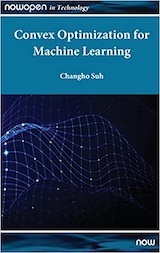Book Description

This book covers an introduction to convex optimization, one of the powerful and tractable optimization problems that can be efficiently solved on a computer. The goal of the book is to help develop a sense of what convex optimization is, and how it can be used in a widening array of practical contexts with a particular emphasis on machine learning.

• Dr. Changho Suh is an Associate Professor of Electrical Engineering at Korea Advanced Institute of Science and Technology (KAIST).
Reviews, Ratings, and Recommendations: Related Book Categories: Read and Download Links:Similar Books:
•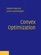Convex Optimization (Stephen Boyd, et al.)

On recognizing convex optimization problems and then finding the most appropriate technique for solving them. It contains many worked examples in fields such as engineering, computer science, mathematics, statistics, finance, and economics.

•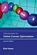Introduction to Online Convex Optimization (Elad Hazan)

This book presents a robust machine learning approach that contains elements of mathematical optimization, game theory, and learning theory: an optimization method that learns from experience as more aspects of the problem are observed.

•Convex Bodies and Algebraic Geometry: Theory of Toric Varieties

This book is an accessible introduction to current algebraic geometry of toric varieties gives new insight into continued fractions as well as their higher-dimensional analogues, the isoperimetric problem and other questions on convex bodies.

•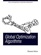Global Optimization Algorithms - Theory and Application

This book is devoted to global optimization algorithms, which are methods to find optimal solutions for given problems. It especially focuses on Evolutionary Computation by discussing evolutionary algorithms, genetic algorithms, Genetic Programming, etc.

•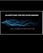Algorithms for Decision Making (Mykel Kochenderfer, et al)

This textbook provides a broad introduction to algorithms for decision making under uncertainty, covering the underlying mathematical problem formulations and the algorithms for solving them.

•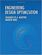Engineering Design Optimization (Joaquim R. Martins, et al)

The philosophy of this book is to provide a detailed enough explanation and analysis of optimization methods so that readers can implement a basic working version. Practical tips are included for common issues encountered in practical engineering design optimization.

Book Categories
 :All CategoriesRecent BooksMiscellaneous BooksComputer LanguagesComputer ScienceData Science/DatabasesElectrical EngineeringJava and Java EE (J2EE)Linux and UnixMathematicsMicrosoft and .NETMobile ComputingNetworking and CommunicationsSoftware EngineeringSpecial TopicsWeb Programming
Other Categories